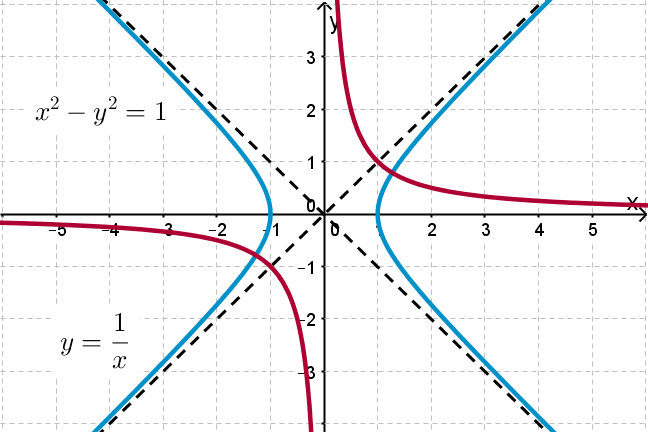1.6

## UNSW SydneyWhat do these 2 hyperbolas have in common?

# Two classical hyperbolas in the Cartesian plane

The seemingly simple hyperbola $\normalsize{y=1/x}$ has many interesting aspects. It is our basic model for an inverse relation, and it embodies the fundamental arithmetical operation of taking the reciprocal of a number. It has a sister hyperbola with equation $\normalsize{x^2-y^2=1}$ that features prominently in Einstein’s special theory of relativity.

In this step we

• look at the geometry of these two curves

• find equations for secants and tangents for $\normalsize{y=1/x}$, pleasantly without calculus

## The hyperbola $\normalsize{y=1/x}$

Here is the graph of the deceptively simple relation $\normalsize{y=1/x}$, or equivalently $\normalsize{xy=1}$, which connects a number $\normalsize{x}$ to its reciprocal $\normalsize{1/x}$. A typical point on the curve is $\normalsize{A=[t,1/t]}$, such as $\normalsize [1,1]$, $\normalsize [2,1/2]$ and $\normalsize [3,1/3]$.Note that the equation $\normalsize{xy=0}$ expresses the two asymptotes $\normalsize{y=0}$ and $\normalsize{x=0}$.

## The hyperbola $\normalsize{x^2-y^2=1}$

Here is another hyperbola: with equation $\normalsize{x^2-y^2=1}$. This does not define a function in the usual sense, because it cannot be expressed as a function $\normalsize{y=f(x)}$. Instead this is an example of a more general relation between $\normalsize{x}$ and $\normalsize{y}$.Note that the equation $\normalsize{x^2 - y^2 = 0}$ expresses the two asymptotes $\normalsize{y=x}$ and $\normalsize{y=-x}$ because $\normalsize{x^2 - y^2 = (x-y)(x+y)}$.

Q1 (C): Can you see any similarities between the hyperbolas $\normalsize{y=1/x}$ and $\normalsize{x^2-y^2=1}$? Is there any way to get one from another?

## Finding secants to $\normalsize{y=1/x}$

You’ll recall that any line that intersects a curve in 2 places is called a secant line. Finding the general equation for a secant line through our hyperbola $\normalsize{y=1/x}$ will help us to find a general equation for a tangent line to $\normalsize{y=1/x}$ in the next step.

Suppose that $\normalsize{A=[t,1/t]}$ and $\normalsize{B=[u,1/u]}$ are two points on the hyperbola. To find the equation of the secant line $\normalsize{AB}$ we can first find the slope

and then write the equation as

for some unknown number $\normalsize{b}$.

When we substitute the point $\normalsize{A=[t,1/t]}$ into this equation, we get

so that

It follows that the equation of the secant line is

This can be rewritten also in the form

Q2 (E): What are the $\normalsize{x}$ and $\normalsize{y}$-intercepts of the secant line?

## Tangents to the hyperbola $\normalsize{y=1/x}$

A tangent line intersects a curve at only one point. So, to find the tangent line to the hyperbola at the point $\normalsize{A=[t,1/t]}$ we can just set $\normalsize{t=u}$ in the equation of the secant line. This gives

or, equivalently

Hidden in this seemingly innocent derivation is a key formula of calculus: that the slope of the tangent at the point $\normalsize A=[t,1/t]$ is $\normalsize - \frac{1}{t^2}$, which is the derivative of $\normalsize y=\frac{1}{x}$ at the point $\normalsize x=t$.Q3 (E): What are the $\normalsize{x}$ and $\normalsize{y}$-intercepts of the tangent line?

Q4 (M): Show that the product of the $\normalsize{x}$ and $\normalsize{y}$-intercepts of the tangent line is constant, independent of the point $\normalsize{A=[t,1/t]}$.

Q5 (M): Show that if $\normalsize{P}$ is the point $\normalsize{[2t,0]}$ and $\normalsize{R=[0,2/t]}$ then $\normalsize{Q( A,P)=Q(A,R)}$, where $A$ is the point on the hyperbola. This verifies another of Apollonius’ claims.

A2. Setting $\normalsize{x=0}$ we find the $\normalsize{y}$-intercept is $\normalsize{\frac{t+u}{tu}}.$ Setting $\normalsize{y=0}$ we find the $\normalsize{x}$-intercept is $\normalsize{t+u}.$

A3. From the equation of the tangent line $\normalsize{y=-\frac{1}{t^2}x + \frac{2}{t} }$ we find that the $\normalsize{y}$-intercept is $\normalsize{2/t}$ and the $\normalsize{x}$-intercept is $\normalsize{2t}$.

A4. From the previous example we have that the intercepts are $\normalsize{2/t}$ and $\normalsize{2t}$, and so their product is $\normalsize{2/t \times 2t= 4}$, which is constant.

A5. The quadrance between the points $\normalsize A=[t,1/t]$ and $\normalsize P=[2t,0]$ is

The quadrance between the points $\normalsize A$ and $\normalsize R=[0,2/t]$ is

So $\normalsize Q( A,P)=Q( A,R).$